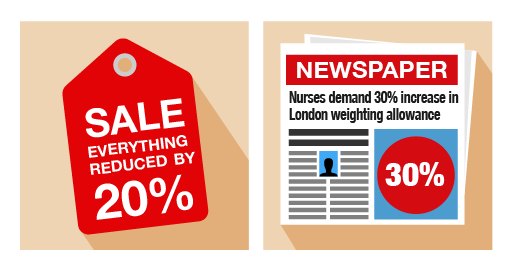Everyday maths 1 (Wales)

Start this free course now. Just create an account and sign in. Enrol and complete the course for a free statement of participation or digital badge if available.

Free course

# 5.1 Percentage increases and decreases

You’ll often see percentage increases and decreases in sales and pay rises.Figure 28 Increasing and decreasing percentages

## Example: Anjali’s pay rise

Anjali earns £18 000 per year. She is given a 10% pay rise. How much does she now earn?

### Method

In order to identify Anjali’s new salary, you need to find out what 10% () of £18 000 is. To do this, first you need to find out 1% () of £18 000:

18 000 ÷ 100 = 180

So 10% () of £18 000 is:

10 × 180 = 1 800

Alternatively, you could find 10% by dividing £18 000 by 10:

18 000 ÷ 10 = 1 800

Anjali’s pay rise is £1 800, so her new salary is:

£18 000 + £1 800 = £19 800

## Example: A sale at the furniture shop

A furniture shop reduces all of its prices by 20%. How much does a £300 double bed cost in the sale?

### Method

In order to identify the new price of the double bed, you need to find out what 20% () of £300 is. To do this, first you need to find out 1% () of £300:

300 ÷ 100 = 3

So 20% () of £300 is:

20 × 3 = 60

The discount is £60, so the sale price of the double bed is:

£300 – £60 = £240

Alternatively, you could find 20% of £300 by dividing by 10 (to find 10%) and then multiplying by 2:

300 ÷ 10 = 30

30 × 2 = 60

## Activity 35: Calculating percentage increases and decreases

1. You buy a car for £9 000. Its value depreciates (decreases) by 25% annually. How much will the car be worth at the end of the first year?
2. Since the start of the 21st century, the shares in the InstaBank have risen by 30%. If the price of one share was £10 in 2000, what is it worth now?
3. The same diamond ring is being sold at different prices, and with different percentage discounts, in two different shops. Which shop offers the better deal?Figure 29 Comparing percentage discounts

1. In order to identify how much the value of the car will decrease by, you need to find out what 25% () of £9 000 is. You can work this out in several different ways. Find 1% () first:
• 9 000 ÷ 100 = 90
Then find 25% () by multiplying by 25:
• 25 × 90 = 2 250
Alternatively, you could have found 25% of £9 000 by dividing 9 000 by 4 (25% = ):
• 9 000 ÷ 4 = 2 250
• The car’s value depreciates by £2 250 in the first year, so the value of the car at the end of the first year will be:
• £9 000 – £2 250 = £6 750
1. It might be easier in this example to convert £10 into pence (£10 = 1 000p). In order to identify the new value of the share, you need to find out what 30% () of 1 000p is. To do this, first you need to find out 1% () of 1 000p:
• 1 000 ÷ 100 = 10
• So 30% () of 1 000p is:
• 30 × 10 = 300
So the price of one share has increased by 300p (£3.00), so the one share is now worth:
• £10 + £3 = £13
Alternatively, you could have found 10% by dividing by 10:
• 1 000 ÷ 10 = 100
You would then multiply by 3 to find 30%:
• 3 × 100 = 300
1. In order to identify Shop A’s discount, you need to find out what 25% () of £500 is. To do this, first you need to find out 1% () of £500:
• 500 ÷ 100 = 5
So 25% () of £500 is:
• 5 × 25 = 125
The discount is £125, so you would have to pay:
• £500 – £125 = £375
You may have worked out 25% of £500 differently. Because 25% is the same as , you may have divided by 4:
• 500 ÷ 4 = 125
Alternatively, you could have halved and halved again:
• 500 ÷ 2 = 250
• 250 ÷ 2 = 125
In order to identify Shop B’s discount, you need to find out what 10% () of £400 is. To do this, first you need to find out 1% () of £400:
• 400 ÷ 100 = 4
So 10% () of £400 is:
• 4 × 10 = 40
The discount is £40, so you would have to pay:
• £400 – £40 = £360
You may have found 10% of £400 by dividing by 10:
• 400 ÷ 10 = 40
Whichever method you used, Shop B offers the best deal.

## Summary

In this section you have learned how to calculate percentage increases and decreases. This will be useful when working out the value of a pay increase or how much an item will cost in a sale. You have also seen that there are different ways of working out percentages. You need to use the method that works for you.You may use different methods for working out different percentages.

FSM_1_CYMRU#### Live in Wales? Free your ambition with a paid part-time course with the OU in Wales.

With grants of up to £4,500* to help with living costs, and tuition fee loans to cover course fees,
now's the time to take that next step.

#### Find out more

*Eligibility rules apply for financial support.